# The Fascinating Math Powering the COVID-19 Vaccine Trials

## An overview of techniques and math used in vaccine studies

With the COVID-19 pandemic raging, big pharma, small pharma, medium sized pharma — pharmaceutical companies of any size with an idea for a vaccine and the funding to pursue it — are racing to get the vaccine out to the physician’s desk, and to get the world out of its nightmare.

It’s against this backdrop, that the world got a rare look at the intricate workings of the massive COVID-19 vaccine trials being conducted by Moderna, Pfizer and AstraZeneca. In the spirit of openness, and for gaining people’s trust, all three companies have published the “protocol” document that they are following for conducting the trials.

The documents go into considerable detail at explaining how the trial is designed and how it will be conducted, the inclusion and exclusion criteria for participants, the criteria for pausing or halting the trial, and the criteria for declaring a vaccine candidate to be an overwhelming success against COVID-19.

The protocol documents also explain how each one of the three main aspects of a vaccine —

its safety,

its immunogenicity i.e. the nature, degree and duration of immune response generated within the participant, and

its efficacy

— will be analyzed using a set of statistical tools and methods.

In the rest of this article, I will focus on the math and the science that drives vaccine efficacy. I will often refer to the data in Pfizer’s protocol document so that we can get frequent reality checks on our calculations, and see how the math works out in the context of a real-life high-stakes vaccine trail.

# Vaccine Efficacy

Vaccine Efficacy is a measure of how much the vaccine was able to reduce the incidence of the disease in a group of people who are vaccinated as compared to a group who are not vaccinated.

Let us imagine a vaccine study that has recruited 2000 volunteers. We will randomly assign 1000 of these volunteers to the “vaccine” group and remaining 1000 to the “control” or “placebo” group. The vaccine group receives the vaccine shot while the control group is given a placebo (often a saline solution).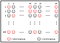1:1 randomized allocation of 2000 participants between the vaccine and control (placebo) groups (Image by Author)

To prevent bias and placebo effects, vaccine studies are conducted in a double-blinded manner i.e. neither the participant nor the person giving the shot knows whether the shot contains the vaccine or a saline solution.

Suppose after receiving the vaccine or placebo injection, all 2000 participants are followed up on for 1 year. In other words, for 2000 “person-years”. During this observation period, suppose 32 of these people develop COVID-19, and out of these 32, 6 are from the vaccine group and 26 are from the control group. I have chosen 32 purposely to match a key number mentioned in Pfizer’s protocol document. More on that as we go along.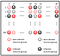Some participants in the vaccine and control groups each, catch COVID-19 during the observation period (Image by Author)

We can calculate the incidence rate of COVID-19 in the vaccine and control groups as follows: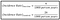Disease incidence rates in the vaccine and control groups (Image by Author)

In general:

Notice that the denominator is in person years, not in persons. Under certain assumptions, 1000 person years’ worth of observational data can be produced by observing 1000 participants for 1 year or 2000 participants for 6 months!

In practice, the actual number of person-years of monitoring will be higher than 1000 to account for participants who are ‘lost to monitoring’, and because one stops monitoring a participant when they develop the disease. So the person years after onset on disease are lost.

Up to a certain point, and under certain assumed risks, the concept of person-years enables the vaccine company to greatly shorten the duration of the trial by simply recruiting a very large number of participants!

For any given person-years’ worth of observations, the Relative Risk (RR) of a participant’s catching COVID-19 after taking the vaccine as compared to not taking the vaccine is as follows:

In our example, RR is calculated as follows:

In the above example, the RR is telling us that the vaccine has the potential to reduce the incidence of disease in a population by 76.9%.

If we calculate RR as a ratio of two incidence rates, the denominator needs to be the same, i.e. same number of person years of monitoring to be used in both vaccine and control groups.

We are now ready to define Vaccine Efficacy (VE). If you had prepared yourself to see a complicated formula, be prepared instead to be pleasantly surprised (or disappointed!) with its anticlimactic simplicity.

For all its simplicity, the Vaccine Efficacy equation has guided the development of modern vaccination and has saved millions of lives.

Before we see how VE is used in vaccine trials, we need to get used to looking at VE in probabilistic terms, in other words, as a random variable. To achieve that perspective, let’s reacquaint ourselves with the constituents of VE, namely the Incidence Rates, but this time we’ll look at Incidence Rates through the lens of chance.

# Modeling the COVID-19 Incidence Rate using the Poisson distribution

A randomly occurring event having a mean rate of occurrence can sometimes be modeled using the Poisson probability distribution, especially when the mean rate equals or is close to its variance.

The number of people catching a disease in a fixed period of time is one such random variable that can be modeled using the Poisson probability distribution (under the mean=variance assumption). Its Probability Mass Function is as follows: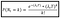Probability Mass Function of a Poisson distributed random variable N_t (Image by Author)

Here, we can consider N_t to be the random variable that represents the number of people catching COVID-19 during time T in the post-vaccination observation period. The above function gives us the probability of k people catching the infection, where k =0,1,2,3,…etc , assuming that λ_t participants catch COVID-19 per unit time. Unit time can be defined as 1 month, 6 months, 1 year, anything we want it to be.

One can see that λ_t is the mean Incidence Rate which we defined earlier. For example, if the mean IR is 16 people per 1000 person years, and we define unit time as 1000 person years, then λ_t=16.

With λ_t=16, the Poisson distributed probability of k trial participants catching COVID-19 during any given block of 1000 person years of observation looks like this:

Notice how the probability peaks around k=16.

Let’s define two more random variables N_c and N_v representing the number of participants in the control and vaccine group respectively who have caught COVID-19 during the observation period. We’ll assume their respective mean IRs as λ_c and λ_v.

Since N_c and N_v are assumed to be Poisson distributed with rates λ_c and λ_v, and N_c and N_v are independent of each other, then by a property of the Poisson distribution, the sum of N_c and N_v is also a Poisson distributed random variable with rate λ_c + λ_v.

But we know that N_t is the sum of N_c and N_v.

λ_t =λ_c + λ_v.

Let’s keep this result in mind for it will be useful to us soon.

For now, the above discussion allows us to define Vaccine Efficacy in terms of two Poisson process rates as follows:

The benefit of expressing the disease incidence as a Poisson distributed random variable is that it allows us to figure out how “powerful” is our statistical testing procedure. Which begs the question, what is meant by the power of a test?

The power of statistical test is a measure of its ability to reject the null hypothesis H0 when the alternate hypothesis H1 is true. It is measured in terms of the probability of rejection of H0 at some desired significance level, when H1 is true.

Before we get any further into explaining how we can define and measure the test’s Power in the context of Vaccine Efficacy, let’s define H0 and H1.

# Null and Alternate Hypothesis for Vaccine Efficacy

Null Hypothesis H0: The “true” Vaccine Efficacy is no greater than some minimum threshold such as 15% or 30%. The low bar on VE is usually set by the vaccine manufacturer.

Alternate Hypothesis H1: The “true” Vaccine Efficacy is greater than the lower threshold.

In their vaccine protocol documents, all three companies: Moderna, Pfizer and AstraZeneca have set the lower threshold on VE to 30%.

At first glance, 30% seems like a low threshold for Vaccine Efficacy. But it is to be interpreted as the lower bound on the confidence interval of the expected true Vaccine Efficacy.

What the Pharma companies are really aiming for is a true VE ≥ 50% with the lower bound on the confidence interval of the estimated VE >30%. This is in line with the recommendation from the US FDA.

But what exactly is 'true VE’?

# What is True VE?

We will use the term True VE in this article a lot. Let’s understand what it means using an example:

Suppose in a randomized trial, the vaccine and the placebo shot are given to 1000 participants each. During the observation period, 50 trial participants have contracted the disease and out these fifty, 7 are from the vaccine group and 43 from the placebo group. Can we conclude that the Vaccine Efficacy is 1-(7/43) = 83.72%?

No, we cannot, because remember that VE is a random variable. If we had the luxury to perform a 2000 person study hundreds of times, then each time we would have seen a different efficacy value. In fact, each one of these hypothetical studies is a 2000 person sample drawn from a population of 7.8 billion (the world’s population in 2020). The True (population level) Vaccine Efficacy will be observed only if all 7.8 billion people are recruited in a study. Since that’s not possible, the best that we can do is assume a suitable probability distribution for the Vaccine Efficacy. Then based on the data observed in only one such 2000 person study, and by using a sound inference mechanism, we conclude that the expected value of True VE must be at least as high as say 60%. We will also be able to show with a certaindegree of confidence (say 95%), that the lower bound on our expectation of True VE is is greater or equal to some lower threshold, say 30%.

The Vaccine Efficacy statistic does a great job of telling us by how much the vaccine has reduced the incidence rate of the disease. But we need something more than a statistic. We need an inference procedure. We need a way to tell us if the point estimate of VE that was observed during the trial can be used to conclude that the True VE is large enough to reject the null hypothesis and thereby declare success.

But how can we tell if the inference procedure isn’t doing a lousy job of inferring the True VE? For instance, how to assess its ability to reject the null hypothesis if the alternate hypothesis is true? We assess this ability using what’s known as the Power of a statistical test.

# Power of the Statistical Test for Vaccine Efficacy

The Power of the statistical test for Vaccine Efficacy is its ability to reject the null hypothesis H0 that the Vaccine is not even minimally effective, when in reality, the true efficacy of the vaccine happens to be greater or equal to the lower threshold.

Notation wise:

(1-β) = P(Reject H0| H1)

Where β is the probability of committing a Type-II error i.e. the probability of declaring the vaccine to be ineffective, when in reality, it happens to satisfy the minimum efficacy criterion.

Let us now convert this equation to a form that is suitable for calculation.

Recollect that H0 says that True VE ≤ some lower threshold VE_LT.

H1 says that True VE > VE_LT.

So we can rewrite the equation for Power like so:

(1-β) = P(Reject H0| True VE > VE_LT)

VE (which we know is a random variable) can be assumed to have some probability distribution. So under that assumption, we would reject H0 at some significance level α, if the following three conditions come true:

1. We have waited until a certain minimum number of participants N_min have caught the disease in the observation period, out of which at most N_vUT are vaccinated participants, AND,
2. We assume that H1 is true, i.e. the true vaccine efficacy is above a certain assumed value such as 60%. In this case, the probability that at most N_vUT vaccinated participants catch the disease out of a total N_min infections, should be at least as high as the desired power level.
3. We assume that H0 is true, i.e. the vaccine is not even minimally effective and True VE ≤ some lower threshold VE_LT such as 30%. In this case, the probability that at most N_vUT vaccinated participants catch the disease out of a total N_min infections, should be at most equal to the desired significance level α (e.g. 0.05).

In notation form, we express #2 and #3 as follows:

The Power of the test can be increased by:

1. Increasing the observed effect size (in our case, the observed efficacy), or,
2. By dialing up the sample size (by recruiting a large number of participants in the trial), or,
3. By setting a large value for the statistical significance criterion (a.k.a. the α-value). But dialing up that 3rd knob, the α-value for accepting H1, comes with the risk of increasing the Type-I error, i.e. risk of declaring an ineffectual vaccine to be an unmitigated success.

# How to Calculate the Values of N_min and N_vUT for a Desired Power Level

In equations 2A and 2B, the term P(N_v ≤ N_vUT) is simply the sum of the probabilities of N_v=k, where k=0,1,2,3,…,N_vUT. Let’s write this statement out as a an equation:

Applying Bayes’ rule to the probability inside the summation:

We can use the fact that N_t = N_c + N_v to rewrite the clause: (N_t=N_min) as (N_c=N_min-k):

Recollect that N_c, N_v and N_t are Poisson distributed random variables with mean incidence rates of λ_c, λ_v and λ_t respectively such that λ_t = λ_c + λ_v. We’ll put these recollections to excellent use.

Let’s use the Poisson Probability Mass Function to express all three probabilities in Eq (5):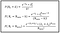Poisson Probability Mass Functions for N_v, N_c and N_t (Image by Author)

Plugging these probabilities into eq (5), and after performing some simplifications, we get the following:

Recollect Eq (1) in which we expressed Vaccine Efficiency in terms of mean disease incidence rates. Let’s reproduce it below for reference and also plug it into Eq (6) so as to express (6) in terms of Vaccine Efficacy: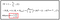Eq (6) expressed in terms of Vaccine Efficacy (Image by Author)

If you have a paper and pen handy, I will encourage you to work out the math and verify that these substitutions actually work to yield equations (6) and (7).

Let’s keep in mind the transformation shown in the red box. It’s a very useful equation that we’ll get to in a bit.

Meanwhile, if the R.H.S. of Eq (7) reminds you of something, you wouldn’t be alone! It’s the Probability Distribution Function (more accurately, the Probability Mass Function) of a Binomially distributed random variable. So, we can re-write Eq (7) like this: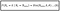Eq (7) expressed using the PMF of a Binomially distributed random variable (Image by Author)

Where Bin(N_min, k, θ) is the probability of seeing k events of interest in N_min trials given that the probability of a single occurrence is θ. But using the Binomial PMF in this manner isn’t going to work out for us unless θ, the probability parameter, is shown to vary smoothly from 0.0 to 1.0. Let’s verify that is indeed the case.

Let’s first look at the endpoints of the scale. VE can take on any value from -∞ to 1.0. In the extreme scenario where everyone from the vaccine group catches the disease and no one from the control group does so, Relative Risk RR → ∞ and VE=1 — RR → -∞. Conversely, if no one from the vaccinated group catches the disease, while everyone from the control group does so, RR = 0.0 and VE is 1.0.

Since θ=(1-VE)/(2-VE), when VE → -∞, θ → 1.0. And when VE=1.0, θ=0

A plot of θ versus VE shows how θ varies smoothly in the open-close interval (1.0, 0], as VE varies smoothly in the open-close interval (-∞, 1]:

In fact, this is exactly the transform function used by the folks at Pfizer in their vaccine protocol document (see page 111).

Let’s plug in Eq (8) into Eq (3):

Finally, let’s plug in the (True VE =VE_assumed) and (True VE = VE_LT) clauses which we had omitted for brevity:

Notice that the R.H.S of 10A and 10B is the Cumulative Distribution Function of the Binomial distribution. We can rewrite Eq(10A) in a concise form as follows:

Where, F(.) is the CDF of the Binomial distribution. Ditto for Eq (10B).

We need to fix certain parameters so that we can calculate the values of N_vUT and N_min for the desired power at a certain significance level α and a lower bound VE_LT on the efficacy.

We’ll use the parameters used by Moderna, Pfizer and AstraZeneca in their respective protocol documents.

All three companies are aiming for a 90% desired power level for their test at a significance level of 0.05 (i.e. 95% confidence level) to conclude that the null hypothesis (VE >= 30%) can be rejected, under the assumption that the true VE is 60%.

So, we will set the following parameter values:

(1-β) = 0.9 , VE_assumed = 60% , θ_assumed = (1–0.6)/(2–0.6) = 0.28571

α=0.05, VE_LT = 30%, θ_0 = (1–0.3)/(2–0.3) = 0.41176

To calculate N_vUT and N_min that will yield the above power under the specified True VE assumption, we will vary N_min from 0 to some arbitrary value (say, 200) in steps of 1. For each value of N_min, we’ll vary N_vUT from 0 to the current value of N_vUT in steps of 1, and we’ll use Equation (10A) to calculate the power. If the calculated power is greater or equal to 0.9, we plug in the combination (N_vUT, N_min) into Equation (10B) to calculate the p-value — the probability of observing the earlier power level under the assumption that in fact the vaccine is not even minimally effective. In other words H0 is true and True VE≤0.3. If this p_value is ≤ the significance level α=0.05, we note down this combination of (N_vUT, N_min) as a tuple of interest.

The above procedure can be easily executed using a simple Python script which I have embedded at the bottom of the article.

When we plot all such selected combinations (N_vUT, N_min), we get the plot shown below (I’ll explain the red marker on the plot in a bit). Meanwhile, notice that we have also varied VE_assumed from 0.3 to 0.9 in steps of 0.1.

We do not see any plots for True_VE=0.3, 0.4 and 0.5 because our procedure was not able to find any (N_vUT, N_min) combinations at these assumed true VE values that would satisfy our desired power level of ≥ 90% at a significance level of 0.05.

One can see that for any given value of observed N_min, desired power level and significance criterion, the max number of infections that one should expect to see in the vaccine group (N_vUT) decreases as the assumed True VE increases.

## The red marker

The red plus marker in the plot marks the data point:

(N_min, N_vUT)=(164, 53)

This is the value used by Pfizer in their protocol document.

Pfizer have assessed (on page 15 of protocol document) that under the assumption that the True VE is 60% and 4 Interim Analyses are performed (more on IA in the next section), observing 164 COVID-19 cases will provide 90% power to reject the Null Hypothesis of True VE >= 30% at a significance level of 95% (i.e. α=0.05).

The fact that the red marker is almost on the True VE=60% step-line in our plot, shows that our analysis appears to have largely borne out the above assessment provided no more than 53 of those 164 cases are found to be in the vaccine group.

We’ll explain the concept of Interim Analysis next.

# Interim Analysis

Vaccine trials can go on for quite some time. The trials being conducted by Moderna, Pfizer and AstraZenecca are expected to go on for at least 24 months. During this period, the participants will be followed up on several times to determine the short, medium, and long-term effects of the vaccine in terms of its safety, immunogenicity, and its efficacy.

However, when there is a severe public health crisis like the COVID-19 pandemic, it helps to get an early indication of vaccine efficacy. The concept of Interim Analysis achieves this objective. With IA, one can get a good estimate of vaccine efficacy when just a small number of participants catch the disease during the observation period.

AstraZeneca’s plan calls for 1 IA when 75 participants catch COVID-19 during the observation period, before the final analysis at 150 cases.

Moderna’s trial plan calls for 2 IAs: The first one when COVID positive case count reaches 53 during the observation period, and the second IA at 106 cases, before the final analysis at 151 cases.

Pfizer’s plan calls for 4 IAs at 32, 62, 92 and 120 positive cases before the final analysis at 164 cases.

As before, we’ll use Pfizer’s example to illustrate how Interim Analysis works.

## The First Interim Analysis (IA1)

Let’s take the example of IA1 in Pfizer’s plan: It is done when 32 participants develop COVID-19 during the observation period. Out of these 26, 6 are from the vaccinated group and 26 are from the control group.

This gives us a point estimate of VE of 1-(6/26) = 0.769. This looks like a good efficacy, but we cannot depend on it too much as it’s a single data point and it may not represent the true population level efficacy. What would really help us to know is the probability of seeing at most 6 cases of COVID-19 in the vaccine group given that the total number of cases observed are 32 and assuming that the true VE ranges from 30% to say 90%.

Equation (9) will give us this information:

We will fix N_min=32, N_vUT= 6. We’ll vary VE_assumed from 0.3 to 0.9 in increments of 0.01 and plot the corresponding probability. We get the following plot: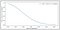P(N_v ≤6 | (N_t=32) for different values of assumed True VE ranging from 0.3 to 0.9 (Image by Author)

If you read off the probability on this plot for values of θ_assumed corresponding to VE_assumed = 30% (i.e. 0.3), 50%, 60%, 70% and 80% (or use equation 10A or 10B to calculate these point estimates), you will get the following values (be sure to transform VE to θ before reading off the probability):

The probabilities in the table above match perfectly with the values mentioned by Pfizer in columns 1 & 2, Table 7, page 113 in their protocol document.

Let’s mark these five point estimates of probability on the above plot: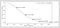The five probability estimates marked up on the plot of P(N_v ≤6 | (N_t=32) for different values of assumed True VE (Image by Author)

Tables 6 and 7 in the Pfizer document also mention the conditional probabilities of observing no more than 15, 25, 35 and finally 53 COVID-19 cases in the vaccine group out of respectively 62, 92, 120 and 164 total cases, at respectively Interim Analysis points 2, 3, 4 and the Final Analysis.

Each one of these probabilities can be calculated using Equation 9 in the same way as for IA1.

Does this mean that one needs to wait until all IAs are completed before considering the vaccine to be an overwhelming success?

Not necessarily, because there is another way to look at the Vaccine Efficacy data, and that is through the lens of a Beta distribution.

# The Beta distribution

The Beta distribution gives you a way to estimate the true probability of an event given a single observation. For example, if you pick up a batch of 100 widgets coming down an assembly line, and find 10 of them to be defective, i.e. a defect rate of 0.1, you can use the Beta distribution to find out the probability (density) of the true defect rate, given this single observation of 0.1.

The Beta distribution is a continuous probability distribution i.e. it applies to continuous random variables such as real numbers (as against discrete ones such as integers).

The Probability Density Function (PDF) of the Beta distribution is as follows:

θ is the random variable in question. In case of the Beta distribution, θ is itself a probability and it represents the true (population) probability of an event, given a single observation.

α and β are parameters that determine the shape of the Beta distribution. Γ(.) is the Gamma function.

The Beta distribution has a Bayesian personality. We initialize it with a couple of prior values of α and β. Typically, we choose α and β that will make the distribution as permissive as possible i.e. we make the least amount of assumptions related to the priors. For example, we could start with α=1 and β=1 signifying that the event is as likely to happen as not to happen. When we see an actual observation of 10 widget failures in a batch of 100 widgets, we update the α and β values as follows to get the revised (posterior) true event probabilities:

α = 1 + number of times the event was observed = 1 + 10 = 11

β = 1 + number of times the event was not observed = 1 + 90 = 91

Next, we recalculate the expected value of the true (population) probability θ of the event in question using the above PDF.

Following is the probability density of the Beta distribution for α=11 and β=91: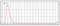PDF of the Beta distribution with α=11 and β=91 (source: desmos.com. Non-commercial use)

The expected value of θ is:

E(θ) = α/(α+β) = 11/(11+91) = 0.1078. This is the the expected value of the true failure rate given a single observation.

Notice that the PDF plot shows us that there are other possible values of the true probability also, each having a different probability density.

What does all this mean for vaccine trials?

# Applicability of the Beta distribution to vaccine trials

Suppose at the first Interim Analysis point, we have observed a point estimate of Vaccine Efficacy as 1-(6/26) = 0.769. That is when a total of 32 people are observed to fall sick with COVID-19 in the observation period and 6 out of those 32 were from the vaccine group.

We want to use the Beta distribution to estimate the expected value of True VE given this single observation of 6 out of 32. To do so, we need to use the random variable θ of the Beta distribution which ranges from 0.0 to 1.0 to represent the Vaccine Efficacy which ranges from -∞ to 1.0. Fortunately, we already have this transformation in place from our earlier work on Power analysis:

θ = (1-VE)/(2-VE)

We also need to ‘prime’ the Beta distribution with the priors for α and β. Let’s use the priors that Pfizer has used in their protocol document (page 111, 7.5 Interim Analyses):

α = 0.700102, and β = 1

These priors are permissive in that they leave a considerable room for improvement in the expected True VE when additional evidence of COVID-19 cases is encountered in the observation period.

The corresponding expected value of θ is as follows: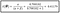Expected value of θ using α = 0.700102, and β = 1 (Image by Author)

This corresponds to an expected True VE of: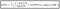Expected value of a Beta distributed True VE corresponding to α = 0.700102, and β = 1 (Image by Author)

30% is also the lower bound on True VE that we want to work with.

It’s no coincidence that Pfizer has set the priors for α = 0.700102, and β = 1 to these specific values.

Suppose we meet the criteria for IA1 when a total of 32 participants develop COVID-19 during the observation period and up to 6 of those 32 are from the vaccine group.

Let’s use this evidence to update α and β:

α = 0.700102 + 6 = 6. 700102

β = 1 + 26 = 27

Following figure shows the updated probability density function of θ: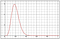PDF of the Beta distribution with α=6. 700102 and β=27 (source: desmos.com. Non-commercial use)

The updated expectation of θ is as follow:

E(θ) = 6. 700102/(6.700102+27) = 0.19881

Which corresponds to an updated expectation for the True Vaccine Efficacy of:

E(True VE) = (1–2*0.19881)/(1– 0.19881) = 0.7518 = 75.18%

An expectation of 75% for the true Vaccine Efficacy is very good indeed.

We can use the Cumulative Probability Distribution (CDF) of the above Beta distribution to know the probability of θ being less than a certain value. Since θ is related to VE in an inverse kind of way, the CDF will also give us the probability of observing an expected value of Vaccine Efficacy that is greater than a certain value.

Let’s illustrate this on the PDF of Beta (6. 700102, 27) using a expected True VE of 30%, which corresponds to θ = 0.41176:

P(θ ≤0.41176) = 0.99648

This probability is the area of the shaded region of the PDF curve shown below:

Since P(θ ≤0.41176) = 0.99648 corresponds to an expected True VE >= 30%, we have made a significant finding:

P(True VE >= 30% | data observed in IA1) = 0.99648

Thus, under the assumptions of a Beta distributed Vaccine Efficacy and with the assumed priors, we can conclude with a near 100% probability that the expected population Vaccine Efficacy will be at least 30% if we find that 32 people catching COVID-19 during the observation period with no more than 6 of those 32 being from the vaccine group.

However, and this is a BIG However, data gathered from subsequent Interim Analyses may either buttress or dilute the probability of True VE being greater or equal to 30%. That’s just the way the Beta distribution works. Additional evidence will either strengthen or dilute our initial claim.

We can perform this analysis for other values of expected true VE also as shown in the table below:

# Conclusion

In this article, I have tried to bring out some of the interesting math that underlies vaccine trials, especially in the context of the high-stakes COVID-19 vaccine trials that are currently underway. The protocol documents published by the pharmaceutical companies have also given us a valuable window into how the math works out in the context of real-life vaccine trials. And when the situation demands it, how math can be used to enormously speedup a trial.

There is plenty more math to dig into in the three protocol documents beyond what has been presented in this article. Happy exploring!

## COVID-19 Vaccine study protocols and US FDA guidance

US FDA guidance: U.S. Department of Health and Human Services, Food and Drug Administration, Center for Biologics Evaluation and Research, June 2020, Development and Licensure of Vaccines to Prevent COVID-19: Guidance for Industry

The source paper for the method of power analysis described in this article: Chan I. S. F., Bohidar N. R., Exact power and sample size for vaccine
efficacy studies
. Comm. in Stat.-Theory and Methods 27:6,1305-
1322, 1998.

For an excellent explanation of the Exact method propounded in Chan and Bohidar, plus SAS code for those who prefer SAS instead of Python: Loiacono, Matthew M (2018) Sample size estimation and power calculations for vaccine efficacy trials for exceedingly rare diseases. Master’s Thesis, University of Pittsburgh.

Written by

Written by

## Sachin Date

#### In-depth explanations of regression and time series models. Get the intuition behind the equations.